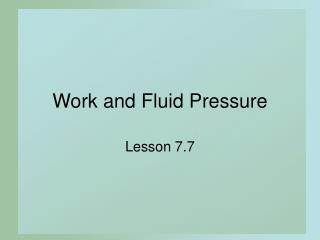Download PresentationWork and Fluid PressureWork and Fluid Pressure - PowerPoint PPT Presentation

Download PresentationWork and Fluid Pressure
An Image/Link below is provided (as is) to download presentation

Download Policy: Content on the Website is provided to you AS IS for your information and personal use and may not be sold / licensed / shared on other websites without getting consent from its author. While downloading, if for some reason you are not able to download a presentation, the publisher may have deleted the file from their server.

- - - - - - - - - - - - - - - - - - - - - - - - - - - E N D - - - - - - - - - - - - - - - - - - - - - - - - - - -
Presentation Transcript

1. Work and Fluid Pressure Lesson 7.7

2. 50 Work • DefinitionThe product of • The force exerted on an object • The distance the object is moved by the force • When a force of 50 lbs is exerted to move an object 12 ft. • 600 ft. lbs. of work is done 12 ft

3. a b x Hooke's Law • Consider the work done to stretch a spring • Force required is proportional to distance • When k is constant of proportionality • Force to move dist x = k • x = F(x) • Force required to move through i thinterval, x • W = F(xi) x

4. Hooke's Law • We sum those values using the definite integral • The work done by a continuous force F(x) • Directed along the x-axis • From x = a to x = b

5. Hooke's Law • A spring is stretched 15 cm by a force of 4.5 N • How much work is needed to stretch the spring 50 cm? • What is F(x) the force function? • Work done?

6. Winding Cable • Consider a cable being wound up by a winch • Cable is 50 ft long • 2 lb/ft • How much work to wind in 20 ft? • Think about winding in y amt • y units from the top  50 – y ft hanging • dist = y • force required (weight) =2(50 – y)

7. Pumping Liquids • Consider the work needed to pump a liquid into or out of a tank • Basic concept: Work = weight x dist moved • For each V of liquid • Determine weight • Determine dist moved • Take summation (integral)

8. r b a Pumping Liquids – Guidelines • Draw a picture with thecoordinate system • Determine mass of thinhorizontal slab of liquid • Find expression for work needed to lift this slab to its destination • Integrate expression from bottom of liquid to the top

9. Pumping Liquids 4 • Suppose tank has • r = 4 • height = 8 • filled with petroleum (54.8 lb/ft3) • What is work done to pump oil over top • Disk weight? • Distance moved? • Integral? 8 (8 – y)

10. Fluid Pressure • Consider the pressure of fluidagainst the side surface of the container • Pressure at a point • Density x g x depth • Pressure for a horizontal slice • Density x g x depth x Area • Total force

11. 2.5 - y Fluid Pressure • The tank has cross sectionof a trapazoid • Filled to 2.5 ft with water • Water is 62.4 lbs/ft3 • Function of edge • Length of strip • Depth of strip • Integral (-4,2.5) (4,2.5) (2,0) (-2,0) y = 1.25x – 2.5x = 0.8y + 2 2 (0.8y + 2) 2.5 - y

12. Assignment • Lesson 7.7a • Page 307 • Exercises 1 – 13 odd, 21 • Lesson 7.7b • Page 307 • Exercises 23 – 35 odd